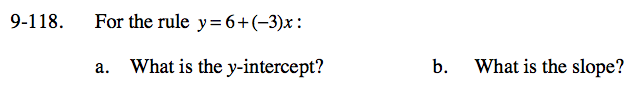### Home > CC3 > Chapter Ch9 > Lesson 9.2.4 > Problem9-118

9-118.
1. For the rule y = 6 + (−3)x: Homework Help ✎

1. What is the y-intercept?

2. What is the slope?The y-intercept is the point at which the graph intersects the
y-axis. The graph will intersect the y-axis when x = 0.

Substitute 0 for x in the expression.
y = 6 + (−3)(0)

y = 6

The slope is the value that y increases or decreases when x increases by one.

Create a table of values or sketch the graph on graph paper.

You should notice that from x = 0 to x = 1 the y-value decreases by 3. What is the slope?